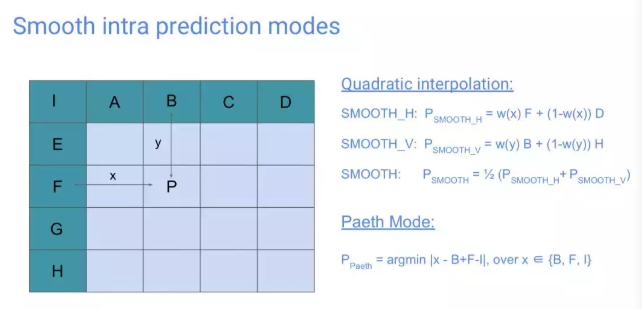# AV1(DAV1D) 解码详解(六)之帧内编码 Intra Prediction

VP9 支持 10 种 帧内预测模式，包括 8 个方向的模式，对应到 45-207 的角度，以及 2 个非方向的预测模式（DC 和 True Motion 模式）。AV1 中，帧内编码器从多个角度进行了扩展：角度预测的粒度进行了升级、利用亮度和色度信号的相干性等等。

AV1 中的帧内预测器，有一种称之为基于递归滤波的帧内预测器。该帧内预测器利用滤波的方式递归地对每个像素值进行预测，在编码方面复杂度会有所提升。

## 1. SPEC Recursive Filtering 概述

The inputs to this process are:
1. a variable w specifying the width of the region to be predicted.
2. a variable h specifying the height of the region to be predicted.
The output of this process is a 2D array named pred containing the intra predicted samples.
For each block of 4x2 samples, this process first prepares an array p of 7 neighboring samples, and then produces the output block by filtering this array.
The variable w4 is set equal to w » 2.
The variable h2 is set equal to h » 1.
The following steps apply of i2 = 0…h2 - 1, for j4 = 0…w4 - 1:
The array p is derived as follows for i = 0…6:
1. If i is less than 5, p[i] is derived as follows:
1. If i2 is equal to 0, p[i] is set equal to AboveRow[(j4 « 2) + i -1].
2. Otherwise, if j4 is equal to 0 and i is equal to 0, p[i] is set equal to LeftCol[(i2 « 1) - 1].
3. Otherwise, p[i] is set equal to pred[(i2 « 1) - 1][(j4 « 2) + i - 1].
2. Otherwise (i is greater than or equal to 5), p[i] is derived as follows:
1. If j4 is equal to 0, p[i] is set equal to LeftCol[(i2 « 1) + i - 5].
2. Otherwise(j4 is not equal to 0), p[i] is set equal to pred[(i2 « 1) + i - 5][(j4 « 2) - 1]
The following steps apply for i1 = 0..1, j1 = 0..3:
1. The variable pr is set equal to 0.
2. The variable pr is incremented by Intra_Filter_Taps[filter_intra_mode][(i1 « 2) + j1][i] * p[i] for i = 0…6
3. pred[(i2 « 1) + i1][(j4 « 2) + j1] is set equal to Clip1(Round2Signed(pr, INTRA_FILTER_SCALE_BITS)).

The output of the process is the array pred.

### DAV1D 中 Recursive Filtering 代码

Dav1d 中关于此预测模式的代码如下：

## 2. Smooth Intra Prediction 概述

### DAV1D 中 Smooth Intra Prediction 代码

3 种平滑预测的方法如下图所示。## 3. Intra Block Copy 概述

AV1 允许它的帧内编码器在预测当前块时，反向搜索当前帧中之前已经重建的部分，该方式在某种程度上与帧间编码搜索之前的帧是类似的。 该方法对于屏幕内容的视频压缩非常有效，因为屏幕内容的视频通常会在同一帧中包含相同的文本、字符等内容。

### IntraBC 几个概念

AV1 SPEC 中关于 IntraBC 的几点。

allow_intrabc equal to 1 indicates that intra block copy may be used in this frame. allow_intrabc equal to 0 indicates that intra block copy is not allowed in this frame.
Note: intra block copy is only allowed in intra frames, and disables all loop filtering. force_integer_mv will be equal to 1 for intra frames, so only integer offsets are allowed in block copy mode.
force_integer_mv equal to 1 specifies that motion vectors will always be integers. force_integer_mv equal to 0 specifies that motion vectors can contain fractional bits.

allow_intrabc 为1，表示该帧中可能存在 intra block copy，否则不存在。同时，intra block copy 仅仅在帧内编码图像中允许，使用了 intra block copy 的块，禁止任何的滤波。

use_intrabc equal to 1 specifies that intra block copy should be used for this block. use_intrabc equal to 0 specifies that intra block copy should not be used.

use_intrabc 为 1，表示该宏块使用 intra_block_copy ，否则不使用该方法。

## 5. Chroma From Luma 概述

AV1 在帧编码中使用了Chroma_From_Luma 的工具，它是利用视觉图像中亮度值和色度值具有高度相似性的特点，通过选择适当的参数结合重建亮度值来预测色度值，该工具在游戏视频中具有很好地压缩效果。

Chrom_From_Luma(CFL) 的整个流程可以由下图表示。1. Compute Luma AC Contribution。
2. Scale Chroma Plane